# Icecreams

Karthik bought 30 icecreams for his birthday .2/5 of them are chocolate icecreams. Find the number of chocolate icecreams he bought?

n =  12

### Step-by-step explanation: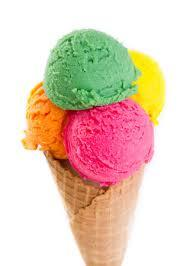Did you find an error or inaccuracy? Feel free to write us. Thank you!Tips to related online calculators
Need help to calculate sum, simplify or multiply fractions? Try our fraction calculator.

## Related math problems and questions:

• Chocolate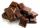Leslie bought 8 same chocolates for 16 Eur. How many euros will he pay for 25 chocolates?Peter bought 1/2 a pound of chocolate at rocky mountain chocolate factory. Later he went to the sweet shoppie and he bought 6/9 of a pound more chocolate. How much chocolate did he buy that day?
• SchoolThere are 150 pupils in grade 5 . 2/3 of them are female. By what fractions are the males?
• Two pizzasJacobs mom bought two whole pizzas. He ate 2/10 of the pizza and his dad ate 1 1/5. How much is left.
• Ten fractionsWrite ten fractions between 1/3 and 2/3
• ComplaintsThe table is given: days complaints 0-4 2 5-9 4 10-14 8 15-19 6 20-24 4 25-29 3 30-34 3 1.1 What percentage of complaints were resolved within 2weeks? 1.2 calculate the mean number of days to resolve these complaints. 1.3 calculate the modal number of day
• Standard deviationFind standard deviation for dataset (grouped data): Age (years) No. Of Persons 0-10 15 10-20 15 20-30 23 30-40 22 40-50 25 50-60 10 60-70 5 70-80 10
• Cake fractions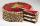Thomas ate 1/3 of cake, Bohouš of the rest of the cake ate 2/5. What fraction of cake left over for others?
• Walking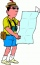The boy walked about 8.5 km in an hour. How long will it take to walk a distance of 32 km if he takes two breaks of 30 minutes during the route?
• 30 eggs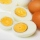There are 30 eggs in a tray. If 1/2 of the tray used 1/5 of it cooked,1/3 kept the refrigerator, how many eggs were left?
• Francesca 2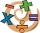Francesca applied the steps below to find the product of (-1.2)(-9.4). Step 1: (-1.2)(-9.4) = (-9.4)(-1.2) Step 2:                   = (-9.4)(-1) + (-9.4)(-0.2) Step 3:                   = (9.4) + (1.88) Step 4:                   = 11.28 Which step shows
• Written numberPlace+values x ten thousands =30 thousands
• Supplementary angles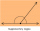(a + 30)° and (2a)° are the measure of two supplementary angles. Find the value of 'a'.
• Consider 2Consider the following formula: y = 3 ( x + 5 ) ( x - 2 ) Which of the following formulas is equivalent to this one? A. Y=3x2+9x-30 B. Y=x2+3x-10 C. Y=3x2+3x-10 D. Y=3x2+3x-30
• FractionsSort fractions z1 = (6)/(11); z2 = (10)/(21); z3 = (19)/(22) by its size. Result write as three serial numbers 1,2,3.
• Reminder and quotientThere are given numbers A = 135, B = 315. Find the smallest natural number R greater than 1 so that the proportions R:A, R:B are with the remainder 1.
• Stamps 2Dennis spent 34.15 on stamps. The number of .56 is 10 less than four times of stamps bought for .41. How many of each stamp did he buy?